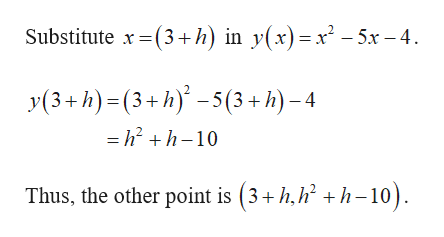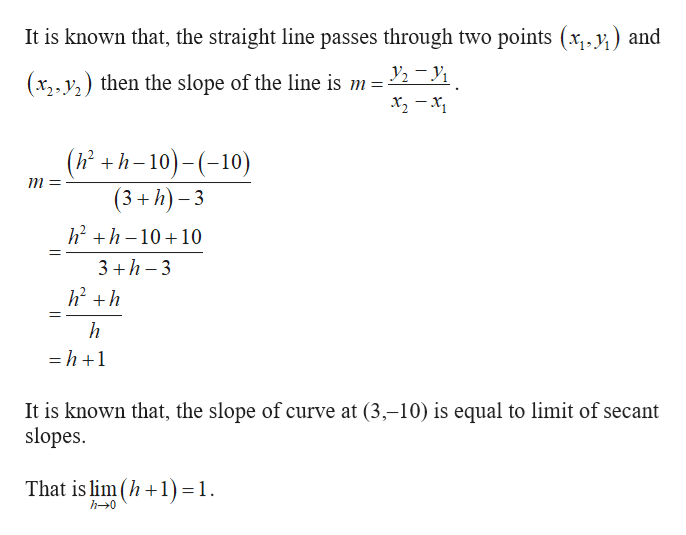Find the slope of the curve y=x2-5x-4 at the point P(3,-10) by finding the limit of the secant slopes through point P. Can you please show me how to solve?

Question

Find the slope of the curve y=x2-5x-4 at the point P(3,-10) by finding the limit of the secant slopes through point P. Can you please show me how to solve?

Step 1

The given equation is y(x) = x2 – 5x – 4 and the given point is (3,–10).

Let the other point of intersection of the secant and the curve be

(3 + h, y (3 + h)).help_outlineImage TranscriptioncloseSubstitute x(3+h) in y(x)=x-5x-4 y(3+h)(3+h)-5(3+h)-4 - h2 +h-10 Thus, the other point is (3+h,h h-10) fullscreen
Step 2help_outlineImage TranscriptioncloseIt is known that, the straight line passes through two points (x,.y) and y2-y1 (x2 y then the slope of the line is m = (h+h-10)-(10) (3+h)-3 m= h2h1010 3 h 3 h -h+1 It is known that, the slope of curve at (3,-10) is equal to limit of secant slopes That is lim(h1) 1 h-0 fullscreen

Want to see the full answer?

See Solution

Want to see this answer and more?

Our solutions are written by experts, many with advanced degrees, and available 24/7

See Solution
Tagged in

Other